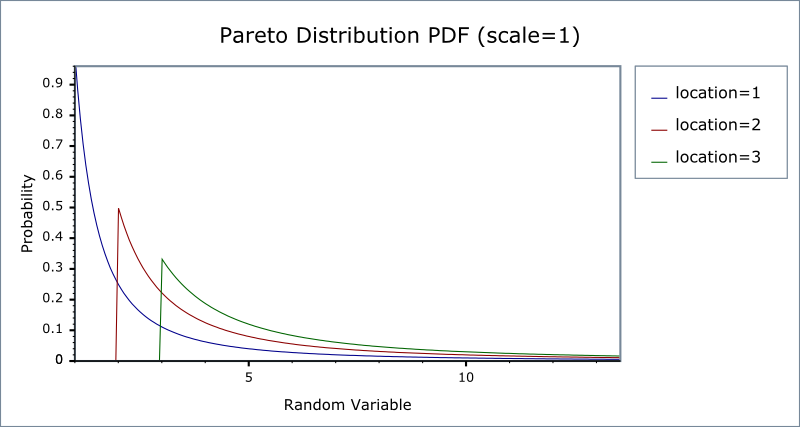#Boost C++ Libraries

...one of the most highly regarded and expertly designed C++ library projects in the world.

This is the documentation for an old version of boost. Click here for the latest Boost documentation.
##### Pareto Distribution

`#include <boost/math/distributions/pareto.hpp>`

```namespace boost{ namespace math{

template <class RealType = double,
class Policy   = policies::policy<> >
class pareto_distribution;

typedef pareto_distribution<> pareto;

template <class RealType, class Policy>
class pareto_distribution
{
public:
typedef RealType value_type;
// Constructor:
pareto_distribution(RealType location = 1, RealType shape = 1)
// Accessors:
RealType location()const;
RealType shape()const;
};

}} // namespaces
```

The Pareto distribution is a continuous distribution with the probability density function (pdf):

f(x; α, β) = αβα / xα+ 1

For shape parameter α > 0, and location parameter β > 0, and α > 0.

The Pareto distribution often describes the larger compared to the smaller. A classic example is that 80% of the wealth is owned by 20% of the population.

The following graph illustrates how the PDF varies with the location parameter β:And this graph illustrates how the PDF varies with the shape parameter α:##### Member Functions
```pareto_distribution(RealType location = 1, RealType shape = 1);
```

Constructs a pareto distribution with shape shape and scale scale.

Requires that the shape and scale parameters are both greater than zero, otherwise calls domain_error.

```RealType location()const;
```

Returns the location parameter of this distribution.

```RealType shape()const;
```

Returns the shape parameter of this distribution.

##### Non-member Accessors

All the usual non-member accessor functions that are generic to all distributions are supported: Cumulative Distribution Function, Probability Density Function, Quantile, Hazard Function, Cumulative Hazard Function, mean, median, mode, variance, standard deviation, skewness, kurtosis, kurtosis_excess, range and support.

The supported domain of the random variable is [location, ∞].

##### Accuracy

The pareto distribution is implemented in terms of the standard library `exp` functions plus expm1 and as such should have very low error rates except when probability is very close to unity.

##### Implementation

In the following table α is the shape parameter of the distribution, and β is its location parameter, x is the random variate, p is the probability and its complement q = 1-p.

Function

Implementation Notes

pdf

Using the relation: pdf p = αβα/xα +1

cdf

Using the relation: cdf p = 1 - (β / x)α

cdf complement

Using the relation: q = 1 - p = -(β / x)α

quantile

Using the relation: x = α / (1 - p)1/β

quantile from the complement

Using the relation: x = α / (q)1/β

mean

αβ / (β - 1)

variance

βα2 / (β - 1)2 (β - 2)

mode

α

skewness

kurtosis

kurtosis excess

##### References
 Copyright © 2006 , 2007, 2008 John Maddock, Paul A. Bristow, Hubert Holin, Xiaogang Zhang and Bruno Lalande Distributed under the Boost Software License, Version 1.0. (See accompanying file LICENSE_1_0.txt or copy at http://www.boost.org/LICENSE_1_0.txt)